# Introduction to GRASS GIS and Scripting in GRASS using Python

## FOSS4G-PH 2016 workshop (April 22, 2016)

MH 210, College of Engineering, University of the Philippines Diliman, Quezon City 1191

Engr. Ben Hur S. Pintor

https://github.com/bnhr07b## WORKSHOP: Clear-sky Solar Radiation using GRASS and r.sun

Software:
Data:
• Region 03 SRTM DEM
• Region 03 Provinces shapefile
• Region 03 Linke turbidity csv
The data can be found here
The Coordinate System to be used is WGS84 UTM Zone 51N (EPSG:32651)

The scripts in the Python tab can be used to run the commands in the Python shell but first you must import the Python Scripting Library using:
``````
import grass.script as gscript
```
```

### Running GRASS and setting up the LOCATION and MAPSET

``````
grass
```
```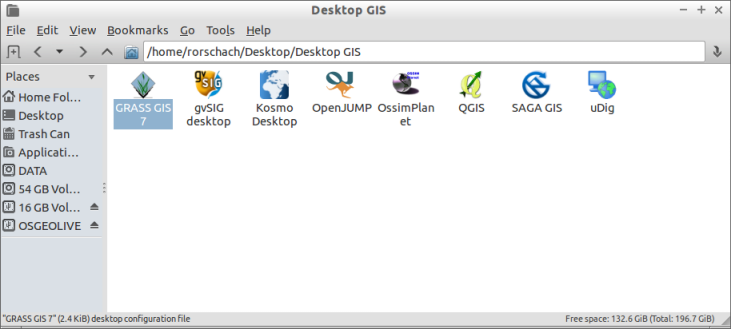```
```

#### LOCATION

1. Create a NEW LOCATION
``````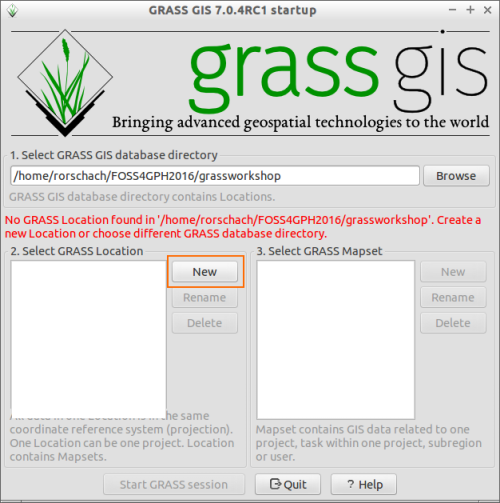```
```
2. Name your LOCATION anything you want (ex. PHL-32651, PHL with UTM Zone 51N CRS)
``````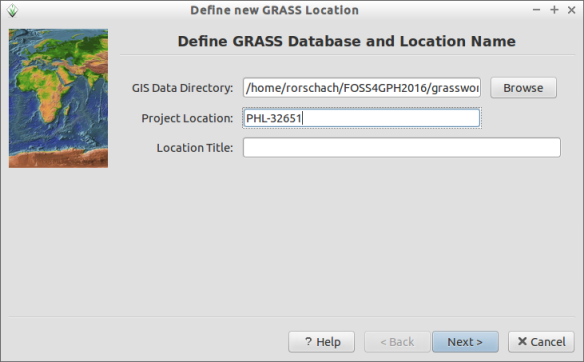```
```
3. Select the first option (Select EPSG Code...)
``````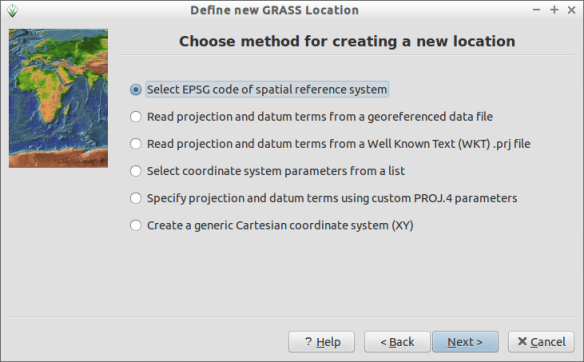```
```
4. Input 32651 (WGS84 UTM Zone 51N)
``````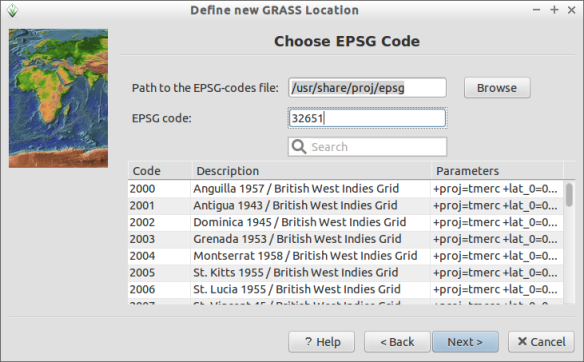```
```
``````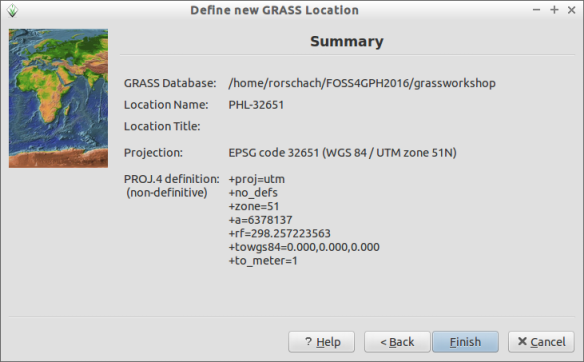```
```

#### MAPSET

1. Create new MAPSET
``````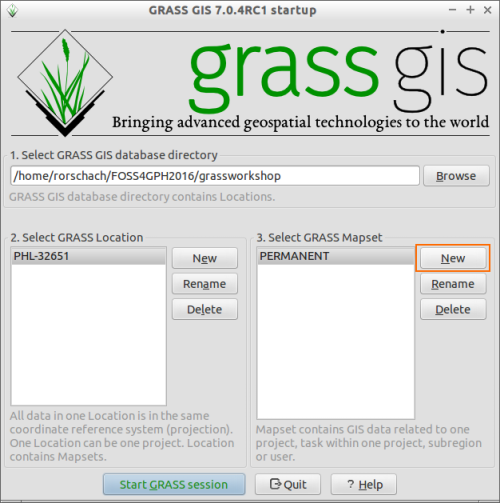```
```
``````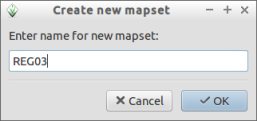```
```
2. Start GRASS Session
``````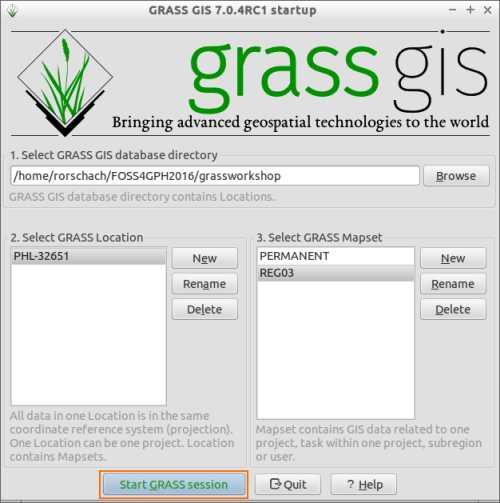```
```
Creating and Editing a LOCATION and MAPSET can also be done within GRASS
``````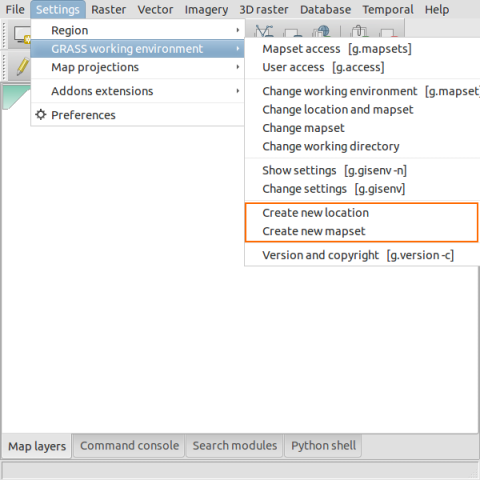```
```

### Pre-processing

#### Importing the elevation raster

``````
r.in.gdal input=path/to/REG03_SRTM90.tif output=elev
```
```
``````

```
```

#### Importing the provinces shapefile

``````
v.in.ogr input=path/to/REG03_provinces.shp output=provinces
```
```
``````

```
```

#### Importing the Linke csv to vector file

The Linke turbidity csv file is formatted as Latitude, Longitude, Linke for January. The Latitude and Longitude values are in WGS84.
##### Convert Coordinates (m.proj)
In order to import the values as a vector in our LOCATION, we must first convert the Latitude and Longitude values to the LOCATION's Coordinate Reference System.
``````
m.proj -i --verbose input=/path/to/REG03_Linke.csv output=/path/to/outputfile separator=comma
```
```
``````

```
```
##### Importing a csv as vector (v.in.ascii)
We then import the output of m.proj using v.in.ascii.
``````
v.in.ascii --verbose input=/path/to/REG03_Linke_proj.csv output=linke separator=comma columns="X DOUBLE PRECISION,Y DOUBLE PRECISION,Jan DOUBLE PRECISION"
```
```
``````

```
```

#### Setting the Computational Region

Before doing any processing, especially for rasters, it is important to make sure that the computational region (extent and resolution) is correct.
You can show the current computational region by:
``````
g.region -ap
```
```
For our purpose, we need to set the computational region equal to that of the elevation raster. This can be done by:
``````
g.region [email protected]
```
```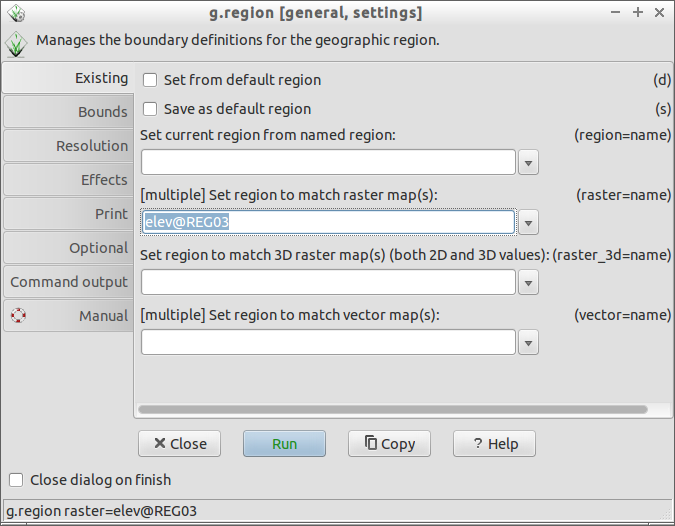```
```

Or by right clicking on the layer and selecting
``````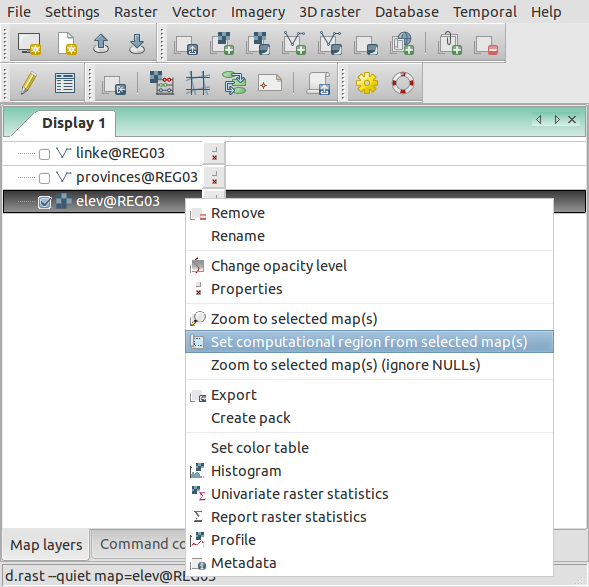```
```

### Processing

#### Slope and Aspect raster

Slope and aspect rasters can be computed using r.slope.aspect
``````
r.slope.aspect elevation=elev slope=slope aspect=aspect
```
```
``````

```
```

#### Horizon raster

Horizon rasters are computed using r.horizon.
For quicker computations, set the computational region to 300 prior to computing the horizon rasters by using:
``````
g.region res=300
```
```
Afterwards, the horizon rasters can be computed by:
``````
r.horizon [email protected] step=15 output=horizon maxdistance=10000
```
```
``````

```
```
Afterwards, make sure to set the computational region back to that of the elevation raster.
``````
g.region [email protected]
```
```

#### Interpolating the Linke turbidity vector

Before interpolating the Linke turbidity vector, we must get the normalized Linke turbidity value using the formula: Ln = L + (0.00035 * elevation).
The first step is to add columns to hold the elevation and normalized Linke values at the vector points.
``````
v.db.addcolumn [email protected] columns="Elev DOUBLE PRECISION,Jan_norm DOUBLE PRECISION"
```
```
``````

```
```
This can also be done by right clicking on the vector -> Show attribute data -> Manage Tables -> Add columns
``````

```
```
##### Sample elevation values at the vector points (v.what.rast)
The next step is to get the elevation values at the vector points. This can be done by sampling the elevation raster at the vector points with v.what.rast.
``````
v.what.rast [email protected] [email protected] column=elev
```
```
``````

```
```
##### Compute for the normalized Linke values (v.db.update)
The Jan_norm field can be updated using:
``````
v.db.update --verbose [email protected] layer=1 column=Jan_norm query_column="Jan + (0.00035 * elev)"```
```
``````

```
```
##### Interpolating for the January Linke turbidity raster using Regularized Spline with Tension (v.surf.rst)
``````
v.surf.rst --verbose [email protected] zcolumn=Jan_norm elevation=linke_Jan [email protected] tension=100 smooth=0
```
```
``````

```
```

#### Clear-sky solar radiation computations using r.sun

r.sun is a topography based solar radiation model implemented in GRASS GIS whose main inputs are an elevation raster and the Julian date. Here we will use r.sun to compute for the clear-sky GHI on January 1 of Region 3. The inputs that we will use are:
• Elevation raster (elev)
• Slope raster (slope)
• Aspect raster (aspect)
• Horizon rasters (horizon)
• Angle step = 15
• Julian date = 1 (January 1)
``````
```
```
``````

```
```

### Post-processing

#### Changing the colors of the raster (r.color)

Changing the color scheme of rasters can be done using r.color. Built-in color rules are provided but users can also create their own color rules.
For example:
``````

```
```
You can also save the color table to a file so that you can re-use it.

Zonal statistics like getting the average raster values inside a polygon can be done using v.rast.stats. Here, we want to compute for the average clear-sky solar radiation received by each province for the day (Jan 1).

First we load the provinces vector into the Layer Manager. Then we use v.rast.stats to compute for the average GHI received by each province.
``````
v.rast.stats --verbose [email protected] [email protected] column_prefix=ghi method=average
```
```
``````

```
```

#### Changing the color of the province based on the solar radiation values (v.colors)

``````

```
```
If the color change doesn't automatically reflect on the Map Window, try to refresh the display by zooming or panning.

### Other common modules/operations

#### Raster Calculations (r.mapcalc)

Let's say we want to get the average of 2 rasters (clear_GHI_001, clear_GHI_002) and save it as clear_GHI_ave. We can use GRASS' r.mapcalc.
``````
r.mapcalc "clear_GHI_ave = ([email protected] + [email protected])/2"
```
```
``````

```
```

#### Removing data from the LOCATION (g.remove)

Make sure that you tick the force removal checkbox and select the data type.
You use regular expressions or wildcards to remove multiple data with the same names.
``````
g.remove
```
```

```
```

#### Resampling a raster (r.resamp.stats)

Before resampling a raster, you should first set the resolution of the computation region to that of the resolution you want to resample to. For example, if you want to resample from 90m to 300m, first set the resolution of the computation region to 300 by:
``````
g.region res=300
```
```

Resampling is done using r.resamp.stats. This resamples the raster based on an aggregation method (e.g. average, median, mode, etc.) chosen by the user.
``````
r.resamp.stats input=raster_to_be_resampled output=resampled_raster
```
```
``````

```
```